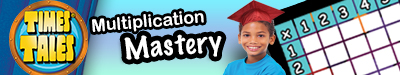## Multiplication Worksheets

Multiplication Worksheets Free and PrintableThese Multiplication PDFs cover 1 Minute Timed, 2-9’s, Word Problems, Input and Output, Regrouping, No Regrouping, Box Puzzles, Multiplying by Fractions, Multiplying by Decimals, Multiplication Tables, and Circle Drill. Find worksheets for basic, intermediate and advanced students that require regrouping and no regrouping. Teachers, parents, and students can print these Multiplication PDFs and make copies.

Get psyched for the most ultimate FREE System to Master Multiplication! Students will use Multiplication Chants, Multiplication Video Flashcards, and Printable PDFs for drills. Go to Rudolph Academy’s FREE System for Mastering Multiplication.

Printable FREE Multiplication Table

Timed 1 Minute Multiplication PDFs – Use these drill worksheets to find out where there is a need for more practice. For example, is the student having problems with Fours, Sixes, Sevens, or Nines Multiplication?
Twos Through Nines Multiplication PDFs
Twos Multiplication PDFs
Threes Multiplication PDFs
Fours Multiplication PDFs
Fives Multiplication PDFs
Sixes Multiplication PDFs
Sevens Multiplication PDFs
Eights Multiplication PDFs
Nines Multiplication PDFs
Basic Multiplication PDFs
100 Problems Multiplication PDFs
Multiplication Word Problems PDFs
Input Output Multiplication PDFs
No Regrouping Multiplication PDFs
Regrouping Multiplication PDFs
Multiplication Box Puzzles PDFs
Multiplying by Fractions PDFs
Multiplying by Decimals PDFs
Multiplication Tables PDFs
Circle Drill Multiplication PDFs

Go to Rudolph Academy FREE Mastering Multiplication SystemGet a Test Overview and 100 FREE Practice Questions for the following GATE Tests!
AABL®     Bracken School Readiness Assessment™ (BSRA™)     CCAT™   CogAT®